# Code for OpenCV cameras to OpenGL cameras.

July 2, 2019

This post offers some code for implementing the information in the previous page of this tutorial, which covered the theory and equations of converting camera calibration information in the OpenCV context to the OpenGL context. There’s a Github repository to support this post, which you can grab here.

## More preliminaries to get to implementation.

Assuming that you have computed your matrices from the previous page, there’s a couple small items remaining to get to an implementation. A reminder that we had:

$\mathbf{x}_{GL} = \mathbf{NDC} \quad \mathbf{K}_{GL} \begin{bmatrix} \begin{matrix} \mathbf{R} \\ \begin{matrix} 0 & 0 & 0\end{matrix} \end{matrix} & \begin{matrix} \mathbf{t} \\ 1 \end{matrix} \end{bmatrix} \mathbf{X}$

The first item is that there is an additional transformation that may rotate and translate the model – this is sometimes called the modelview matrix in OpenGL-speak. I will denote this matrix as $$\mathbf{M}$$, and it is a $$4 \times 4$$ matrix. This matrix may be a Euclidean transformation, a similarity transform, affine, projective, you name it – it should not be non-singular, but there’s nothing preventing you from specifying a non-singular matrix. Given this, we have:

$\mathbf{x}_{GL} = \mathbf{NDC} \; \mathbf{K}_{GL} \begin{bmatrix} \begin{matrix} \mathbf{R} \\ \begin{matrix} 0 & 0 & 0\end{matrix} \end{matrix} & \begin{matrix} \mathbf{t} \\ 1 \end{matrix} \end{bmatrix} \; \mathbf{M} \; \mathbf{X}$

(Reminder – these 3D->3D transformation in projective space are detailed in the H-Z book, and most will be interested a Euclidean transformation, composed of

$\mathbf{M} = \begin{bmatrix} \begin{matrix} \mathbf{R} \\ \begin{matrix} 0 & 0 & 0\end{matrix} \end{matrix} & \begin{matrix} \mathbf{t} \\ 1 \end{matrix} \end{bmatrix}$

or a similarity transform, which scales coordinates in the model file. This is convenient when the coordinates in the model file are in meters, and millimeters are more convenient (or vice versa):

$\mathbf{M} = \begin{bmatrix} \begin{matrix} s\mathbf{R} \\ \begin{matrix} 0 & 0 & 0\end{matrix} \end{matrix} & \begin{matrix} \mathbf{t} \\ 1 \end{matrix} \end{bmatrix}$

where $$s$$ is a scalar.)

The second item is that $$\mathbf{NDC} \quad \mathbf{K}_{GL}$$ is multiplied together and becomes the matrix

intrinsic=$$\mathbf{NDC} \; \mathbf{K}_{GL}$$.

## Where do you put the matrices?

Okay – now for where to put everything. In the main function, we load the matrices into the shader as follows. Here, intrinsic=$$\mathbf{NDC} \; \mathbf{K}_{GL}$$, and extrinsic=$$\begin{bmatrix} \begin{matrix} \mathbf{R} \\ \begin{matrix} 0 & 0 & 0\end{matrix} \end{matrix} & \begin{matrix} \mathbf{t} \\ 1 \end{matrix} \end{bmatrix}$$. model_trans= $$\mathbf{M}$$.

ourShader.setMat4("intrinsic", opengl_intrinsics);
...


Then, within the shader file materials.vs, which is loaded and compiled at runtime, the transformations are used to compute the OpenGL coordinates. Note that if you get error messages when running the program, it is likely that the shader path is incorrect relative to where you are running the program – take a look at the README.

Normal = mat3(transpose(inverse(model_trans))) * aNormal;

FragPos = vec3(intrinsic*extrinsic*model_trans * vec4(aPos, 1.0));

gl_Position = intrinsic * extrinsic * model_trans * vec4(aPos, 1.0);


# Examples of test cases – cube

First, I’ll show a simple example and some examples, to demonstrate how to do some basic troubleshooting. The first object is that of a $$1\times 1 \times 1$$ cube, centered at the origin. Within the Github repository, in folder Data1, is this file, box.ply. I visualize 3D models with Meshlab (free!), though there are many other options.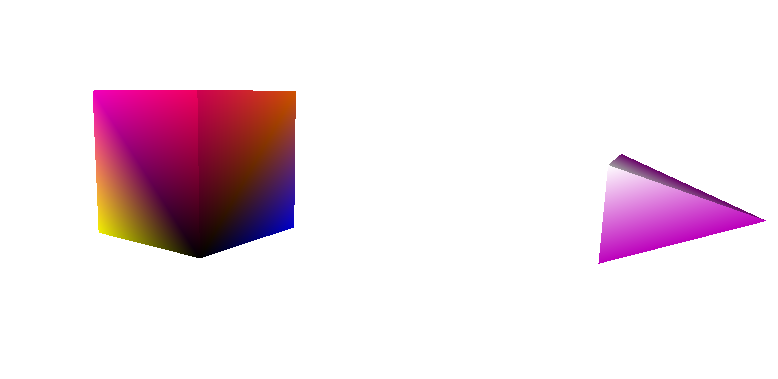Figure 1. A $$1\times 1 \times 1$$ cube, centered at the origin, and representation of a camera, shown by a pyramid. See the text below for more details.

$$\quad$$

Figure 1 shows the cube relative to the camera, represented by a pyramid. The white corner of the pyramid represents the upper left corner of the image plane. If you are viewing the scene in a 3D model viewer, you’ll then be able to rotate the scene so that in if everything is set up, what you see from the view of this pyramid and the captured OpenGL window are similar.

## Input.

The input to the code is a text file with the calibration information in the OpenCV format, as well as some additional information. There are defaults for a lot of this information, but depending on your model and where it is located, if you do not specify the information such that it is relevant, you will be capturing “a whole lot of nothin “, as we say around here.

Here’s an example file with the maximum amount of information:

cols 640
rows 480
K
600       0     320
0 600     240
0       0       1
RTworld2cam
1 0 0 0
0 1 0 0
0 0 1 3
Model
0.70711 -0.70711 0 0
0.70711 0.70711 0 0
0   0 1  0
0   0 0  1
light-position 0 0 -3.5
write-camera 1
camera-scale 0.5
camera-color 170 0 170
shininess 1.0
near 1
far 5
file /home/atabb/git/OpenCV2OpenGL/Data1/box.ply


And explanations:

• rows: image rows
• cols: image cols
• K: $$\mathbf{K}_{CV}$$
• RTworld2cam : $$\mathbf{R} \mid \mathbf{t}$$
• Model: $$\mathbf{M}$$
• light-position: coordinate for the light (I know)
• whether to write a camera .ply file or not
• camera-scale: height of the pyramid representing the camera
• shininess: a lighting parameter, how shiny the material is
• near: distance of the near plane
• far: distance of the far plane
• file: location of the model file. [mandatory]

The only mandatory item is the model file.

Then to call the function, you would do something similar to what is below:

./OpenCV2OpenGL1 --input /home/username/git/OpenCV2OpenGL/Data1/CaliBox.txt --output /home/username/git/OpenCV2OpenGL/Data1/R1 --write-name r1.png --version 0


here,

• input: is the filename for the configuration text file above,
• output: is a directory where a log, image, camera.ply file will be written,
• write-name: is the name you want for the rendered image that is written in the directory,
• version: is 0 for this example. There are other examples in this tutorial that use the same code base.

To quit the program, hit ESC.

## Output.

The code for this stage will output a camera.ply file – if you selected this in the input, as well as a logfile.txt, and an image file with the rendered OpenGL image. One of the arguments to the program is the image’s name – see the README for details on that. To quit the program, hit ESC.

The logfile.txt contains all of the information from your source input file, as well as all of the computed matrices, $$\mathbf{NDC}$$, $$\mathbf{K}_{GL}$$, the camera center, etc.

If you have run the code with your own model and get a blank screen, it is a good idea to visualize the camera relative to your model to make sure the camera’s field of view covers the object. If you are using a model transformation matrix $$\mathbf{M}$$ that is a Euclidean transformation only, an additional camera file called camera-rel-model.ply is written, which provides this visualization.

Another potential issue to check is the near and far planes; any points not contained in between will be clipped. You can use the output contained in the logfile.txt to perform the testing described at the bottom of this section.

The figures below illustrate some basic test cases, which are all included in the Data1 folder of the amy-tabb/OpenCV2OpenGL repository mentioned above. The results generated on my machine are also included in that directory.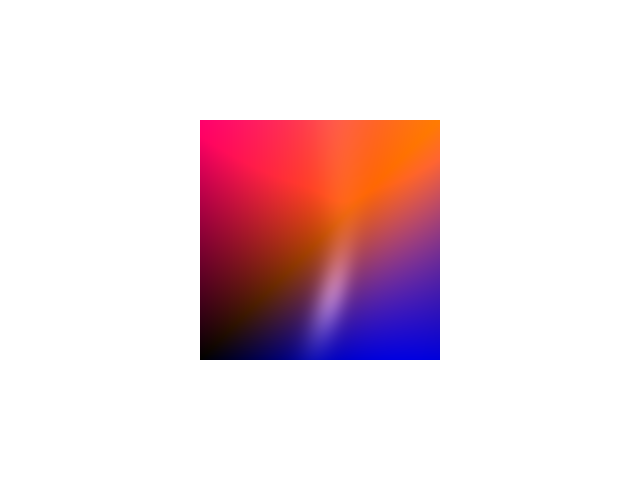$$\;$$ Figure 2. Using the CaliBox.txt file, and results in the R1 directory.

$$\mathbf{K}_{CV} = \begin{bmatrix} \alpha & 0 & \frac{cols}{2} \\ 0 & \alpha & \frac{rows}{2} \\ 0 & 0 & 1 \\ \end{bmatrix}$$

$$\quad$$

Figure 2 shows the result image when using a standard internal camera calibration matrix, where the principal points are in the center of the image. The $$\mathbf{M}$$ matrix is an identity matrix.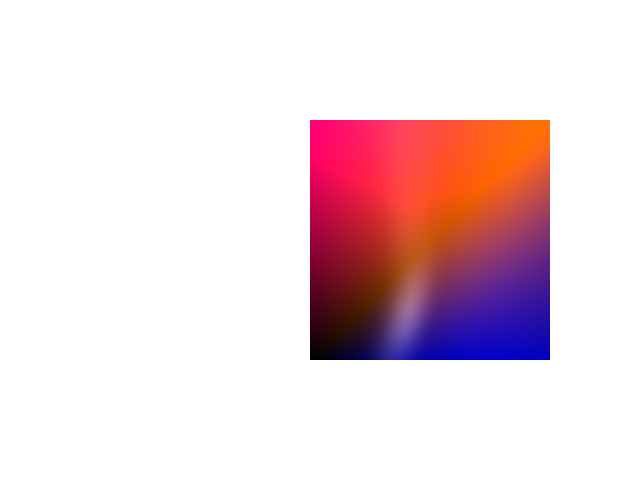$$\;$$ Figure 3. Using the CaliBoxC.txt file, and results in the R2 directory.

$$\mathbf{K}_{CV} = \begin{bmatrix} \alpha & 0 & \frac{cols}{2} + 110 \\ 0 & \alpha & \frac{rows}{2} \\ 0 & 0 & 1 \\ \end{bmatrix}$$

$$\quad$$

To link all of this text to the code and examples, I used similar arguments to generate Figure 3:

./OpenCV2OpenGL1 --input /home/username/git/OpenCV2OpenGL/Data1/CaliBoxC.txt --output /home/username/git/OpenCV2OpenGL/Data1/R2 --write-name r2.png --version 0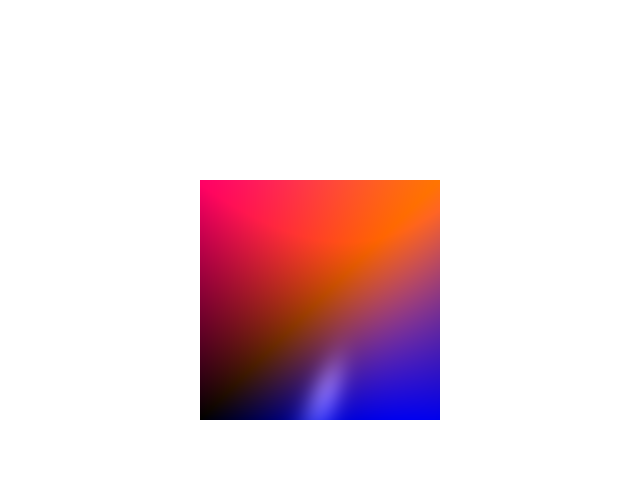$$\;$$ Figure 4. Using the CaliBoxR.txt file, and results in the R3 directory.

$$\mathbf{K}_{CV} = \begin{bmatrix} \alpha & 0 & \frac{cols}{2} \\ 0 & \alpha & \frac{rows}{2} + 60 \\ 0 & 0 & 1 \\ \end{bmatrix}$$

$$\quad$$

These arguments were used to generate Figure 4:

./OpenCV2OpenGL1 --input /home/username/git/OpenCV2OpenGL/Data1/CaliBoxR.txt --output /home/username/git/OpenCV2OpenGL/Data1/R3 --write-name r3.png --version 0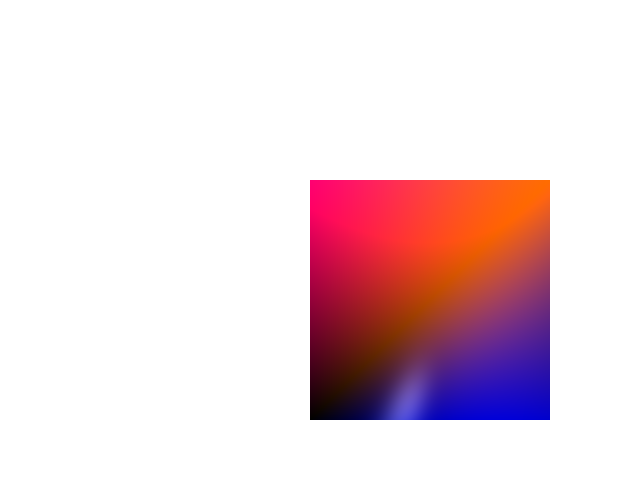$$\;$$ Figure 5. Using the CaliBoxRC.txt file, and results in the R4 directory.

$$\mathbf{K}_{CV} = \begin{bmatrix} \alpha & 0 & \frac{cols}{2} + 110 \\ 0 & \alpha & \frac{rows}{2} + 60 \\ 0 & 0 & 1 \\ \end{bmatrix}$$

$$\quad$$

These arguments were used to generate Figure 5:

./OpenCV2OpenGL1 --input /home/username/git/OpenCV2OpenGL/Data1/CaliBoxRC.txt --output /home/username/git/OpenCV2OpenGL/Data1/R4 --write-name r4.png --version 0


Figures 3-5 vary the principal points, and show the effect of doing so on the rendered image.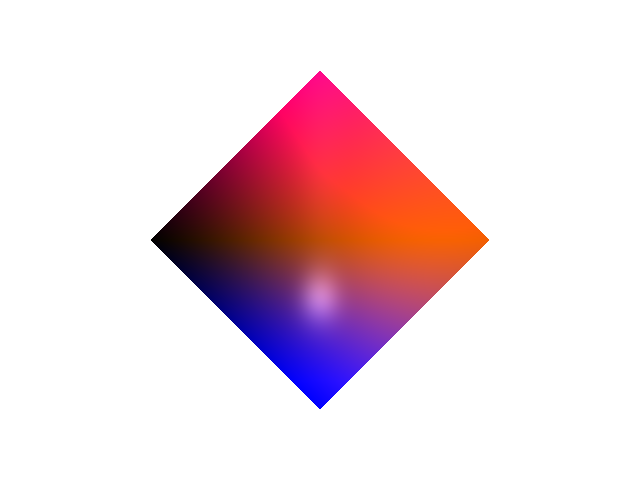$$\;$$ Figure 6. Using the CaliBoxTrans.txt file, and results in the R5 directory.

$$\mathbf{K}_{CV} = \begin{bmatrix} \alpha & 0 & \frac{cols}{2} \\ 0 & \alpha & \frac{rows}{2} \\ 0 & 0 & 1 \\ \end{bmatrix} \quad \mathbf{M} = \begin{bmatrix} 0.70711 & -0.70711 & 0 & 0 \\ 0.70711 & 0.70711 & 0 & 0 \\ 0 & 0 & 1 & 0 \\ 0 & 0 & 0 & 1 \end{bmatrix}$$

$$\quad$$

These arguments were used to generate Figure 4:

./OpenCV2OpenGL1 --input /home/username/git/OpenCV2OpenGL/Data1/CaliBoxTrans.txt --output /home/username/git/OpenCV2OpenGL/Data1/R5 --write-name r3.png --version 0


Figure 6 shows the result when using the internal camera calibration matrix from Figure 2, but then adding a rotation about the $$z$$ axis via $$\mathbf{M}$$.

# Example of test cases - horse.

Here’s a more exciting example. The horse model is from the Georgia Tech Large Model Archive. The number of triangles was reduced, and color was introduced by me, by playing around in Meshlab.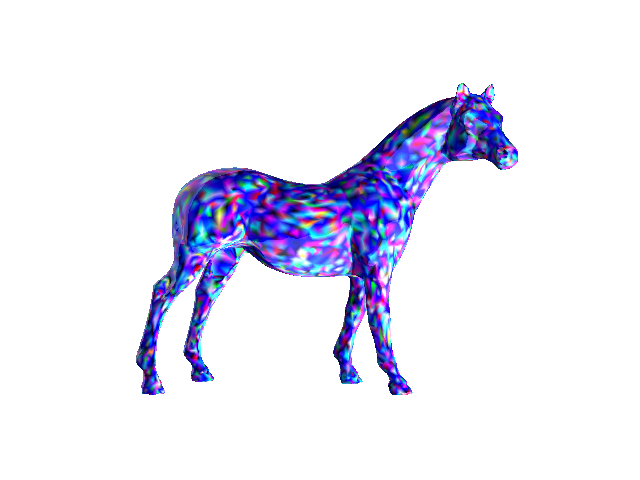$$\;$$ Figure 7. Using the CaliHorse.txt file, and results in the R6 directory.

$$\mathbf{K}_{CV} = \begin{bmatrix} \alpha & 0 & \frac{cols}{2} \\ 0 & \alpha & \frac{rows}{2} \\ 0 & 0 & 1 \\ \end{bmatrix} \quad \mathbf{M} = \begin{bmatrix} 10 & 0 & 0 & 0 \\ 0 & 10 & 0 & 0 \\ 0 & 0 & 10 & 0 \\ 0 & 0 & 0 & 1 \end{bmatrix}$$

$$\quad$$

Here, the I used a similarity transform for the $$\mathbf{M}$$ matrix, since the units on the horse figure are so small. Multiplying by $$\mathbf{M}$$ effectively scales the model by $$10$$.

These arguments were used to generate Figure 7:

./OpenCV2OpenGL1 --input /home/username/git/OpenCV2OpenGL/Data1/CaliHorse.txt --output /home/username/git/OpenCV2OpenGL/Data1/R6 --write-name r1.png --version 0
`

The next post will demonstrate how to generate images of rotating models. I have found these very effective for visualizations of the work.

### Changelog

• Updated with command-line calls for each of the examples, June 2020.

© Amy Tabb 2018 - 2022. All rights reserved. The contents of this site reflect my personal perspectives and not those of any other entity.